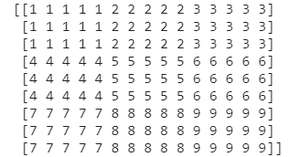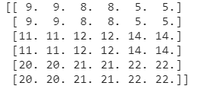# How to upsample a matrix by repeating elements using NumPy in Python?

• Last Updated : 03 Jan, 2021

Prerequisites: Numpy

Upsampling a matrix simply means expanding it and obviously upsampling can be done by adding more elements to the original matrix. It can be done in various ways like adding new elements and expanding the original matrix or it can be done by the matrix elements of original matrix itself. The later approach is discussed below along with 2 methods to do the same.

### Method 1: using repeat()

We use the numpy.repeat() method to upsample the matrix by repeating the numbers of the matrix. We pass the matrix in repeat() method with the axis to upsample the matrix. This method is used to repeat elements of array.

Syntax:

numpy.repeat(array, repeats, axis=0)

Parameters:

• array=Name of the array
• repeats= Numbers of repetitions of every element
• axis= The axis along which to repeat the values. By default, axis is set to None.
• For row-wise axis=0 and for column-wise axis=1.

Approach

• Import module
• Create array
• Pass it to repeat method
• Print matrix

Example:

## Python3

 `# importing required module ` `import` `numpy as np ` ` `  `# declaring an array ` `a ``=` `[[``1``, ``2``, ``3``], [``4``, ``5``, ``6``], [``7``, ``8``, ``9``]] ` ` `  `# use the repeat function to upsample the array ` `print``(np.repeat(a, ``5``, axis``=``1``).repeat(``3``, axis``=``0``)) `

Output:### Method 2:

In this approach we will see how np.kron is used to upsample a matrix. We pass the matrix along with an ones matrix which will multiply with each other using kron() method and the result will be an upsampled matrix.

Syntax:

`np.kron(a ,b)`

where a and b are two arrays.

• It returns the Kronecker product of two arrays.
• Its parameters are two arrays whose product to be calculated

Example:

## Python3

 `# import required libraries ` `import` `numpy as np ` ` `  `# creating an array using numpy ` `a ``=` `np.array([[``9``, ``8``, ``5``], [``11``, ``12``, ``14``], [``20``, ``21``, ``22``]]) ` ` `  `# using kron function upsampling the array ` `upsampled_array ``=` `np.kron(a, np.ones((``2``, ``2``))) ` ` `  `# printing the desired result ` `print``(upsampled_array) `

Output :My Personal Notes arrow_drop_up
Recommended Articles
Page :